# Maxim Addresses High-Voltage Needs in Industrial Ultrasound Applications

### Abstract

Most ICs for ultrasound transmission available today are aimed to cover medical applications and do not necessarily fit the needs for industrial applications. Industrial applications such as nondestructive tests (NDTs), flow meters, and sonar often have different requirements for voltage capability, current capability, and frequency. Thanks to their great flexibility, Maxim's high-voltage (HV) products can be used in a broad range of applications. This article presents a variety of applications for the MAX4940 quad HV digital pulser and the MAX4968 HV multiplexer (mux).

### Overview

#### The MAX4940 HV Digital Pulser

Figure 1 shows the essential functional diagram of the MAX4940, a device that features 4 channels for high-voltage (HV) digital pulsing. (Only 1 out of the 4 channels is displayed in the diagram.)

• S1 and S2 switches (connected to VPP_ and VNN_, respectively) feature 200V voltage capability and 2A current capability.
• S3 switch (called "clamp" in the data sheet) features 200V voltage and 1A current capability.
• The digital pulser can operate in both bipolar and unipolar applications. In other words, all of the following cases are supported:
• [VPP, VNN] = [+100V, -100V] bipolar
• [VPP, VNN] = [0, -200V] unipolar negative
• [VPP, VNN] = [+200V, 0] unipolar positive
• INP_ and INN_ control S1 and S2, respectively.
• INC_ controls S3 (clamp), but it is conditioned by S1 and S2. In most applications, INC_ does not need to be driven. You can always keep it high and drive INP_ and INC_ only. In doing so, S3 is activated every time both S1 and S2 are off.INP_ INN_ CLP_ OUT_ X X X High-Z 0 0 0 High-Z 0 0 1 GND 0 1 X VNN_ 1 0 X VPP_ 1 1 X Not allowed

#### The MAX4968 HV Analog Switch

Figure 2 shows the essential functional diagram of the MAX4968, which features 16 independent HV analog switches. Each switch's internal status can be programmed with an SPI interface. In most ultrasound applications, the HV analog switches are used to implement HV muxes.

• SW1A, SW1B can swing from VNN to VNN + 200V.
• The HV analog switches can operate both in bipolar and unipolar applications. The input/output voltage range can be one of the following cases:
• (SW_) range = [+100V, -100V] bipolar
• (SW_) range = [0, -200V] unipolar negative
• (SW_) range = [+200V, 0] unipolar positive
• VNN can vary from 0V to -200V, depending on the amplitude of the input signal and its polarity. VNN can be shared with the pulser (transmitter) negative supply.
• VPP is a low-voltage supply (10V only)
• Equivalent RON is flat in the entire input range (approximately 20Ω) and on-capacitance is 16pF only.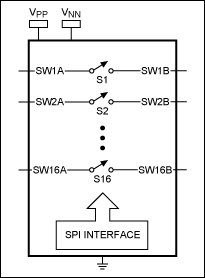### Supported Applications

Maxim's HV pulser and switches are unique in that they can operate in both bipolar and unipolar applications. (Most industrial ultrasound applications are unipolar.) Maxim solutions provide a great benefit both in terms of size reduction and system simplification for unipolar applications. Application diagrams and time charts are shown below for both bipolar and unipolar applications. External grass-clipping diodes can be in some cases omitted.

#### Bipolar Applications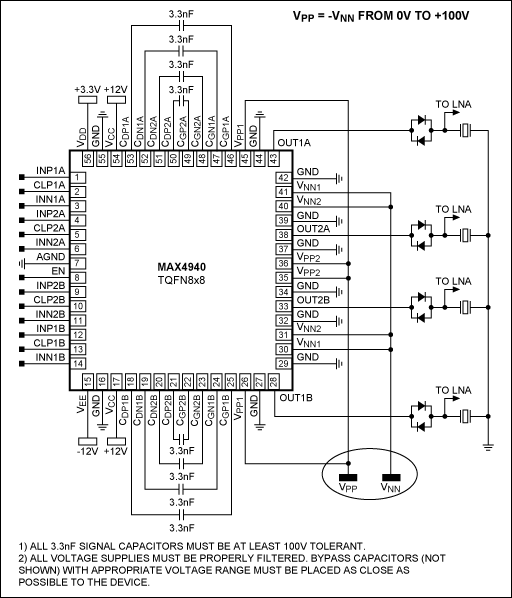Notes

1. For simplicity, only two channels are connected and the MAX4968 is configured as a 1:2 HV mux.
2. Two HV supplies only are required (VPP, VNN).
3. The MAX4940's exposed pad (not shown in Figure 4) must be connected to VNN.Note: CLP_ is always high. Only two control signals per channel for typical 3-level transmission.

#### Unipolar Positive ApplicationsNotes

1. Only one HV supply is required.
2. Notice that CGN_ can be directly connected to CDN_ so that one capacitor per channel is removed.
3. VEE voltage supply for the MAX4940 is no longer needed (VEE pin is grounded).
4. Capacitor tolerance is 200V.
5. The MAX4940's exposed pad (not shown) can be grounded.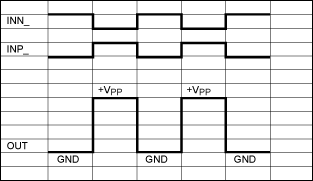#### Unipolar Negative Applications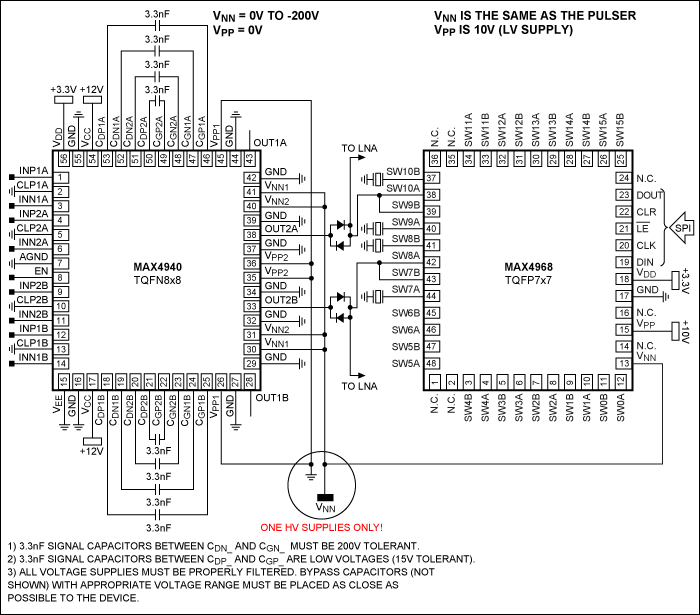Notes

1. One HV supply only is required.
2. The MAX4940's exposed pad (not shown) is connected to VNN.
3. The unipolar positive (Figures 6 and 7) configuration is slightly preferable when compared with the unipolar negative (fewer external components are needed and the exposed pad is connected to GND plane for better thermal performance).### Doubling the Voltage Driver Capability with BTL Configurations

In industrial applications, driving the transducer with voltages greater than 200V is often required. Transducer elements used in NDT, flow metering, or other applications can require more than 200V pulsing for better performance.

The MAX4940 can drive the transducer element in a bridge-tied load (BTL) configuration, resulting in a doubling of the equivalent peak-to-peak excitation signal. Two MAX4940 channels need to be used to drive a single transducer element. The excitation voltage can be up to 400VP-P.

The BTL configuration is possible whenever both electrodes of the single element transducer are available. This does not apply to large transducer arrays in which elements typically share a common node normally connected to GND.

Figure 12 shows a typical BTL application diagram. The starting point is the unipolar configuration (positive in this example). However, the transducer load is now connected between OUT1_ and OUT2_. Figure 13 shows a typical driving scheme. As a result, the amplitude of the excitation signal can reach 2 x VPP, that is 400VP-P.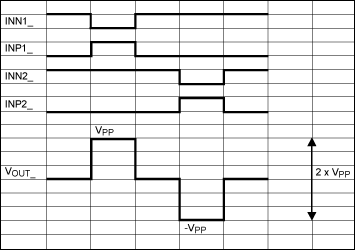### Increasing the Current Driver Capability with Parallelization

In industrial applications, a current driving capability greater than 2A is sometimes required. This high capability can be used to drive a highly capacitive load (in the order of nF) or operate at a high frequency (e.g., up to 30MHz to 40MHz). The MAX4940 channels can be parallelized to increase the current capability (2 channels = 4A, 3 channels = 6A, and so on).

For example, Figure 14 shows a positive unipolar 4A digital pulser using 2 channels in parallel to drive a single element. Although the figure showcases the MAX4940, similar considerations can be done for the MAX4968. Related approaches can be also used in bipolar and unipolar-negative applications. Channels can be parallelized to reduce the on-resistance and increase the driving capability.It is also possible to parallelize channels when using a BTL configuration. In other words, the MAX4940 can be used to drive a single element up to 400VP-P with 4A current capability (Figure 15).#### Low- and High-Frequency Operations

The application diagrams discussed above cover most applications. However, there are cases in which low-frequency signals are used (< 1MHz). This is frequently the case in sonar applications, which can range from 10kHz to 200kHz.

Alternatively, operating at a high frequency (> 20MHz) is sometimes required, often to improve axial resolution in NDT applications or to transmit PWM modulated signals. The sections below illustrate both types of applications.

#### Low Frequency (< 1MHz)

The MAX4940 can operate at a frequency lower than 1MHz. It is sufficient to replace the signal capacitors (3.3nF in the examples above) with capacitors that have greater values. As a rule of thumb, you can consider the following formula:

CSIGNAL = 3.3nF/freq(MHz)

For instance, for a 100kHz application, the suggested value is 33nF. Contrastingly, the MAX4968 is based on a bootstrap architecture. It cannot operate at frequencies lower than 100kHz.

#### High Frequency (> 20MHz)

The driver circuit of the MAX4940 can operate at a high frequency (generating short pulses), such as 40MHz. However, the actual limitation is normally due to the finite driving current capability.

In a first-order approximation, the load can be considered purely capacitive (CLOAD). The maximum slew rate that you can achieve is related to the peak current that the pulser can provide (IPEAK). In formula,

SLEW_RATE = (δV/δt)max = IPEAK/CTOT

Where CTOT is the total load capacitance, including the transducer capacitance, cable capacitance, and IC parasitic capacitance.

Now suppose you are working in unipolar positive mode (Figure 6) and trying to transmit a 200V unipolar burst. If for example CTOT = 400pF, then the slew rate is limited to:

(δV/δt)max = IPEAK/CTOT = 2A/300pF = 6.66V/ns

And consequently, the rise and fall time are approximately given by:

TRISE = TFALL ≈ 200V/(6.66V/ns) = 30ns

Therefore the minimum pulse width for 200V amplitude is approximately 60ns.

Of course, similar consideration can be done for bipolar and unipolar negative applications.

As explained above, you can increase the current capability (IPEAK) by putting more channels in parallel. (Notice that the IC parasitic capacitance will also increase accordingly). Thus, it is possible to increase the operating frequency. For instance, with the 4A pulser shown in Figure 14, you can theoretically (neglecting the IC parasitic capacitance) get TRISE = TFALL = 15ns for a 200V output swing.

IPEAK = 4A, CTOT = 300pF, swing = 200V → minimum pulse width = 30ns

Similar consideration applies to other operating modes, such as bipolar and unipolar negative.